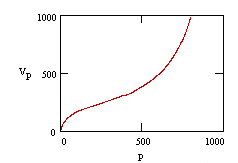# Derivation of the Langmuir isotherm

 For molecules in contact with a solid surface at a fixed temperature, the Langmuir Isotherm, developed by Irving Langmuir in 1916, describes the partitioning between gas phase and adsorbed species as a function of applied pressure.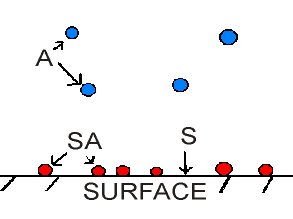## Thermodynamic Derivation

The adsorption process between gas phase molecules, A, vacant surface sites, S, and occupied surface sites, SA, can be represented by the equation,assuming that there are a fixed number of surface sites present on the surface.

An equilibrium constant, K, can be written: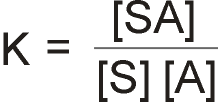q = Fraction of surface sites occupied (0 <q< 1)

Note that

• [SA] is proportional to the surface coverage of adsorbed molecules, or proportional to q
• [S] is proportional to the number of vacant sites, (1 - q)
• [A] is proportional to the pressure of gas, P

Thus it is possible to define the equilibrium constant, b: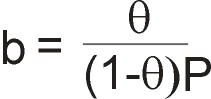Rearranging gives the expression for surface coverage: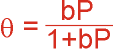### Kinetic Derivation

The rate of adsorption will be proportional to the pressure of the gas and the number of vacant sites for adsorption. If the total number of sites on the surface is N, then the rate of change of the surface coverage due to adsorption is: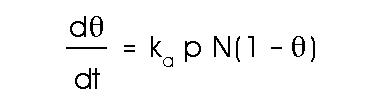The rate of change of the coverage due to the adsorbate leaving the surface (desorption) is proportional to the number of adsorbed species:In these equations, ka and kd are the rate constants for adsorption and desorption repectively, and p is the pressure of the adsorbate gas. At equilibrium, the coverage is independent of time and thus the adorption and desorption rates are equal. The solution to this condition gives us a relation for q:where b = ka/kd.

Dependence of b on external parameters:

b is only a constant if the enthalpy of adsorption is independent of coverage.

As with all chemical equilibria, the position of equilibrium (determined by the value of b) will depend upon a number of factors:

1. The relative stabilities of the adsorbed and gas phase species involved.
2. The temperature of the system (both the gas and surface, although these are normally the same).
3. The pressure of the gas above the surface.
In general, factors (2) and (3) exert opposite effects on the concentration of adsorbed species - that is to say that the surface coverage may be increased by raising the gas pressure but will be reduced if the surface temperature is raised.

## Graphical form of the Langmuir Isotherm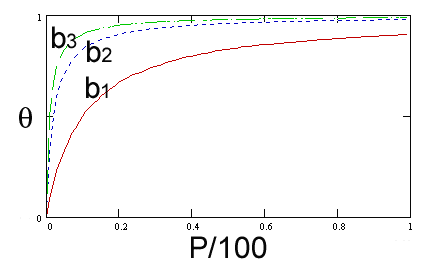where b3 > b2 > b1

# Derivation of the BET Isotherm

Consider a surface: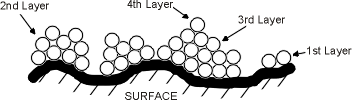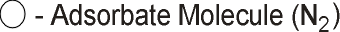Definition:

 q0, q1, ..., qn = Surface area (cm-2) covered by 0, 1, ..., n layers of adsorbed molecules.

At Equilibrium:

 q0 must remain constant.

```        .      Rate of Evaporation        Rate of Condensation
. .                           =
from First Layer          onto Bare Surface
```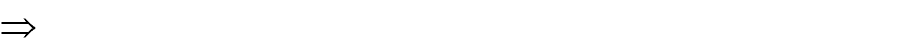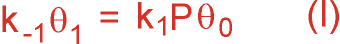Similarly, at equilibrium q1 must remain constant.

 ``` . Rate of Condensation Rate of Condensation . . on the Bare Surface on the 1st Layer + = + Rate of Evaporation Rate of Evaporation from the second layer from the second layer ```
 ``` . . . ``` k1Pq0 + k-2q2 = k2Pq1 + k-1q1

Substituting into (I) gives

k-2q2 = k2Pq1

Extending this argument to other layers,Definitions:

Total surface area of the catalyst,Total volume of gas adsorbed on surfacewhere v0 is the volume of gas adsorbed on one square centimeter of surface when it is covered with a complete layer.

 ``` . . . ```where vm is the volume of gas adsorbed when the entire surface is covered with a complete monolayer.

From (I),If we assume that the properties of the 1st, 2nd, ... layers are equivalent, then,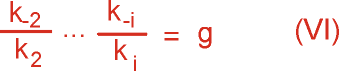Similarly,q3 =xq2 =x2q1

Generally,

 qi =xqi-1 =xi-1q1 =xi-1yq0 =cxiq0 {c=x/y}

Substituting into (V),Now,Also,``` . . . ```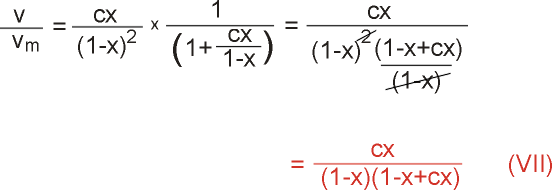At saturation pressure of gas P0, an infinite number of adsorbate layers must build up on the surface. From equation VII, for this to be possible,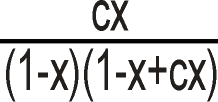must be infinite. This means that at P0, x must equal 1.
 ``` . . . ``` g = P0 (From definition of x)

 ``` . . . ``` x = P/P0

Substituting into VII, we arrive at the recognized form of the BET isotherm,This can be rearranged to give,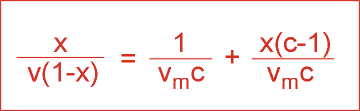## Graphical form of the BET isotherm